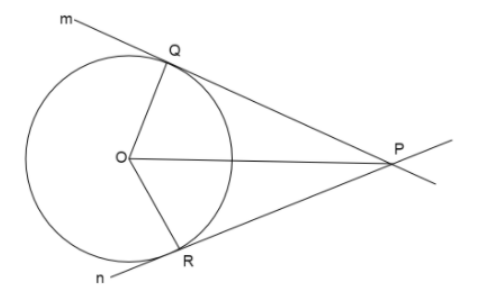Prove that the center of a circle touching two intersecting lines lies on the angle bisector of the lines.Verified
146.4k+ views
Hint: We draw a circle which is touching two intersecting lines. Using the property of tangents from an external point to a circle we prove the two triangles formed by bisectors are congruent by SSS congruence rule.
* Two triangles are said to be congruent to each other by SSS rule if the sides of the first triangle are equal to corresponding sides of the other triangle.
* Two tangents drawn from an exterior point to a circle are equal in lengths.

We draw a circle with center O; point P is the point of intersection of two lines m and n which touch the circle at points Q and R respectively.Here we join the line from center of the circle to the point of intersection of two lines i.e. P.
Now, we know from the property of tangents, that tangents drawn from an exterior point to the circle are equal , so we can say $PQ = PR$
Also, the lengths $OQ = OR$ as they both are the lengths of radius of the circle.
Now, we will consider two triangles $\vartriangle OPQ,\vartriangle OPR$
$OQ = OR$
$PQ = PR$
$OP = OP$
Therefore, by Side Side Side congruence rule, $\vartriangle OPQ \cong \vartriangle OPR$
We know that if two triangles are congruent to each other, then the corresponding angles of two triangles are equal. So, $\vartriangle OPQ \cong \vartriangle OPR$ gives us $\angle OPQ = \angle OPR$.
Therefore we can say that the line $OP$ bisects the angle formed at the intersection of two lines m and n.
Hence Proved

Note: Students make mistake of proving the triangles congruent using SSA congruence as the angles formed by radius and the tangents at points Q and R are right angles, which is wrong because we have no surety of congruence rule SSA, so we cannot use it to prove triangles congruent.